# How to Vlookup multiple values in Excel with criteria

The tutorial shows a few ways to Vlookup multiple matches in Excel based on one or more conditions and return multiple results in a column, row or single cell.

When using Microsoft Excel for data analysis, you may often find yourself in situations when you need to get all matching values for a specific id, name, email address or some other unique identifier. An immediate solution that comes to mind is using the Excel VLOOKUP function, but the problem is that it can only return a single match.

Vlookup for multiple values can be done via a combined use of several functions. If you are far from being an Excel expert, don't hurry to leave this page. I will do my best to explain the underlying logic so that even a novice could understand the formulas and adjust them for solving similar tasks. Even better, I will show you another possible solution that takes just a few mouse clicks and does not require any knowledge of Excel formulas at all!

## How to do multiple Vlookup in Excel using a formula

As mentioned in the beginning of this tutorial, there is no way to make Excel VLOOKUP return multiple values. The task can be accomplished by using the following functions in an array formula:

• IF - evaluates the condition and returns one value if the condition is met, and another value if the condition is not met.
• SMALL - gets the k-th smallest value in the array.
• INDEX - returns an array element based on the row and column numbers you specify.
• ROW - returns the row number.
• COLUMN - returns the column number.
• IFERROR - traps errors.

Below you will find a few examples of such formulas.

### Formula 1. Vlookup multiple matches and return results in a column

Let's say, you have the seller names in column A and the products they sold in column B, column A containing a few occurrences of each seller. Your goal is to get a list of all products sold by a given person. To have it done, please follow these steps:

1. Enter a list of unique names in some empty row, in the same or another worksheet. In this example, the names are input in cells D2:G2: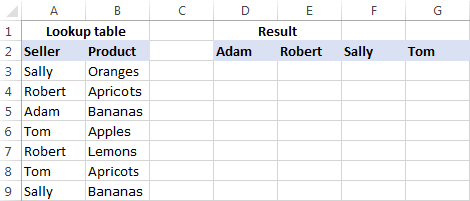Tip. To quickly get all different names in a list, you can use the UNIQUE function in Excel 365 or a more complex formula to extract distinct values in older versions.

2. Under the first name, select a number of empty cells that is equal to or greater than the maximum number of possible matches, enter one of the following array formulas in the formula bar, and press Ctrl + Shift + Enter to complete it (in this case, you will be able to edit the formula only in the entire range where it's entered). Or, you can enter the formula in the first cell, hit Ctrl + Shift + Enter, and then drag the formula down to a few more cells (in this case, you will be able to edit the formula in each cell individually).

`=IFERROR(INDEX(\$B\$3:\$B\$13, SMALL(IF(D\$2=\$A\$3:\$A\$13, ROW(\$B\$3:\$B\$13)-2,""), ROW()-2)),"")`

or

`=IFERROR(INDEX(\$B\$3:\$B\$13,SMALL(IF(D\$2=\$A\$3:\$A\$13,ROW(\$A\$3:\$A\$13)- MIN(ROW(\$A\$3:\$A\$13))+1,""), ROW()-2)),"")`

As you see, the 1st formula is a bit more compact, but the 2nd one is more universal and requires fewer modifications (we will elaborate more on the syntax and logic a bit further).

3. Copy the formula to other columns. For this, select the range of cells where you've just entered the formula, and drag the fill handle (a small square at the lower right-hand corner of the selected range) to the right.

The result will look something similar to this: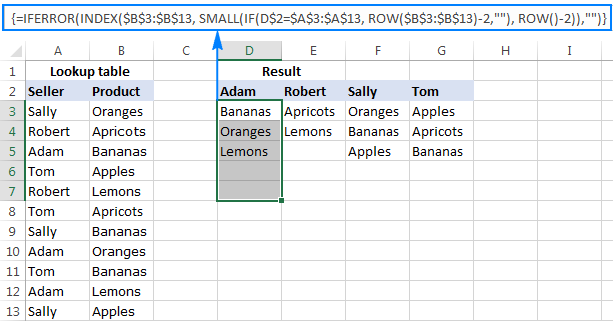#### How this formula works

This is an example of intermediate to advanced uses of Excel that implies basic knowledge of array formulas and Excel functions. Working from the inside out, here's what you do:

1. IF function

At the core of the formula, you use the IF function to get the positions of all occurrences of the lookup value in the lookup range: IF(D\$2=\$A\$3:\$A\$13, ROW(\$B\$3:\$B\$13)-2,"")

IF compares the lookup value (D2) with each value in the lookup range (A3:A13), and if the match if found, returns the relative position of the row; an empty string ("") otherwise.

The relative positions of the rows are calculated by subtracting 2 from ROW(\$B\$3:\$B\$13) so that the first row has position 1. If your return range begins in row 2, then subtract 1, and so on. The result of this operation is the array {1;2;3;4;5;6;7;8;9;10;11}, which goes to the value_if_true argument of the IF function.

Instead of the above calculation, you can use this expression: ROW(lookup_column)- MIN(ROW(lookup_column))+1, which returns the same result but does not require any changes regardless of the return column location. In this example, it'd be ROW(\$A\$3:\$A\$13)- MIN(ROW(\$A\$3:\$A\$13))+1.

So, at this point you have an array consisting of numbers (positions of matches) and empty strings (non-matches). For cell D3 in this example, we have the following array: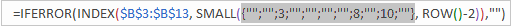If you check with the source data, you will see that "Adam" (lookup value in D2) appears on the 3rd, 8th and 10th positions in the lookup range (A3:A13).

2. SMALL function
Next, the SMALL(array, k) function steps in to determine which of the matches should be returned in a specific cell.

With array already established, let's work out the k argument, i.e. the k-th smallest value to be returned. For this, you make a sort of an "incremental counter" ROW()-n, where "n" is the row number of the first formula cell minus 1. In this example, we entered the formula in cells D3:D7, so ROW()-2 returns "1" for cell D3 (row 3 minus 2), "2" for cell D4 (row 4 minus 2), etc.

As the result, the SMALL function pulls the 1st smallest element of the array in cell D3, the 2nd smallest element in cell D4, and so on. And this transforms the initial long and complex formula into a very simple one, like this: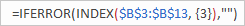Tip. To see the calculated value behind a certain part of the formula, select that part in the formula bar and press F9.

3. INDEX function

This part is easy. You use the INDEX function to return the value of an array element based on its row number.

4. IFERROR function

And finally, you wrap the formula in the IFERROR function to handle possible errors, which are inevitable because you cannot know how many matches will be returned for this or that lookup value, and therefore you copy the formula to a number of cells equal to or greater than the number of possible matches. Not to scare your users with a bundle of errors, simply replace them with an empty string (blank cell).

Note. Please notice the proper use of absolute and relative cell references in the formula. All references are fixed except for the relative column reference in the lookup value (D\$2), which should change based on a relative position of a column(s) where the formula is copied to return matches for other lookup values.

Putting all this together, we get the following generic formulas to Vlookup multiple values in Excel:

Formula 1:

IFERROR(INDEX(return_range, SMALL(IF(lookup_value = lookup_range, ROW(return_range )- m ,""), ROW() - n )),"")

Formula 2:

IFERROR(INDEX(return_range, SMALL(IF(lookup_value = lookup_range , ROW(lookup_range) - MIN(ROW(lookup_range ))+1,""), ROW() - n)),"")

Where:

• m is the row number of the first cell in the return range minus 1.
• n is the row number of the first formula cell minus 1.

Note. In the above example, both n and m are equal to "2" because our return range and formula range both begin in row 3. In your worksheets, these may be different numbers.

### Formula 2. Vlookup multiple matches and return results in a row

In case you want to return multiple values in rows rather than columns, change the above formulas this way:

`=IFERROR(INDEX(\$B\$3:\$B\$13, SMALL(IF(\$D3=\$A\$3:\$A\$13, ROW(\$B\$3:\$B\$13)-2,""), COLUMN()-4)),"")`

Or

`=IFERROR(INDEX(\$B\$3:\$B\$13,SMALL(IF(\$D3=\$A\$3:\$A\$13,ROW(\$A\$3:\$A\$13)- MIN(ROW(\$A\$3:\$A\$13))+1,""),COLUMN()-4)), "")`

Like in the previous example, both are array formulas, so remember to press the Ctrl + Shift + Enter shortcut to complete them correctly.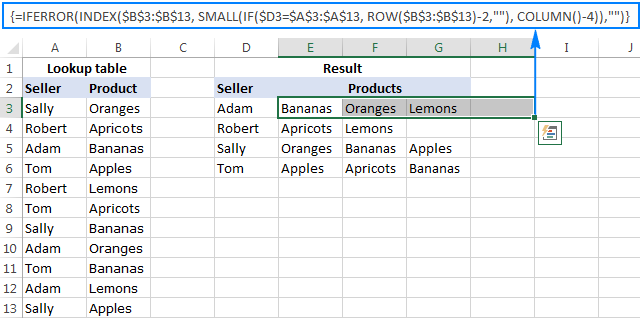The formulas work with the same logic as in the previous example, except that you use the COLUM function instead of ROW to determine which matching value should be returned in a specific cell: COLUMN()-n. Where n is the column number of the first cell where the formula is entered minus 1. In this example, the formula is input in cells E2:H2. With E being the 5th column, n is equal to "4" (5-1=4).

Note. For the formula to get copied correctly to other rows, mind the lookup value references, absolute column and relative row, like \$D3.

Wrapping up, here are the generic formulas for Vlookup with multiple results returned in rows:

Formula 1:

IFERROR(INDEX(return_range, SMALL(IF(lookup_value = lookup_range, ROW(return_range) - m, ""), COLUMN() - n)), "")

Formula 2:

IFERROR(INDEX(return_range, SMALL(IF(lookup_value = lookup_range, ROW(lookup_range) - MIN(ROW( lookup_range))+1,""),COLUMN() - n)), "")

Where:

• m is the row number of the first cell in the return range minus 1.
• n is the column number of the first formula cell minus 1.

### Formula 3. Vlookup multiple matches based on multiple conditions

You already know how to Vlookup for multiple values in Excel based on one condition. But what if you want to return multiple matches based on two or more criteria? Taking the previous examples further, what if you have an additional Month column, and you are looking to get a list of all products sold by a given seller in a specific month?

If you are familiar with arrays formulas, you may remember that they allow using asterisk (*) as the AND operator. So, you can just take the formulas discussed in the two previous examples, and have them check multiple conditions as demonstrated below.

#### Return multiple matches in a column

IFERROR(INDEX(return_range, SMALL(IF(1=((--(lookup_value1=lookup_range1)) * ( --(lookup_value2=lookup_range2))), ROW(return_range)-m,""), ROW()-n)),"")

Where:

• m is the row number of the first cell in the return range minus 1.
• n is the row number of the first formula cell minus 1.

Assuming the Seller list (lookup_range1) is in A3:A30, the Month list (lookup_range2) is in B3:B30, the seller of interest (lookup_value1) is in cell E3, and the month of interest (lookup_value2) is in cell F3, the formula takes the following shape:

`=IFERROR(INDEX(\$C\$3:\$C\$30, SMALL(IF(1=((--(\$E\$3=\$A\$3:\$A\$30)) * (--(\$F\$3=\$B\$3:\$B\$30))), ROW(\$C\$3:\$C\$30)-2,""), ROW()-2)),"")`

This layout may be useful for creating a dashboard, e.g. your users can enter a name in E3, month in F3 and get a list of products in column G: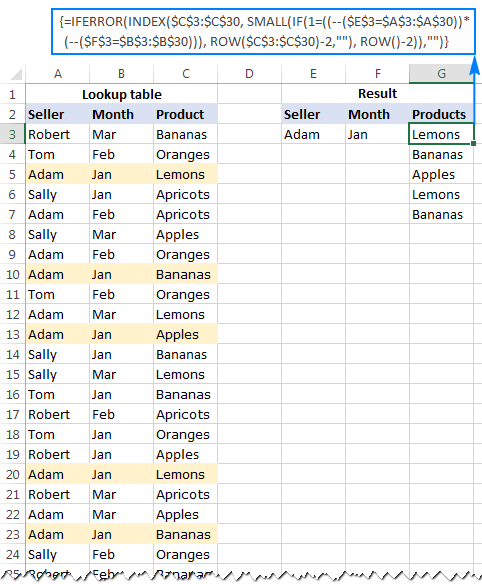#### Return multiple results in a row

If you want to pull multiple values based on multiple criteria sets, you may prefer the horizontal layout where results are returned in rows. In this case, use this following generic formula:

IFERROR(INDEX(return_range, SMALL(IF(1 = ((--(lookup_value1=lookup_range1)) * (--(lookup_value2 = lookup_range2))), ROW(return_range) - m, ""), COLUMN() - n)),"")

Where:

• m is the row number of the first cell in the return range minus 1.
• n is the column number of the first formula cell minus 1.

For our sample dataset, the formula goes as follows:

`=IFERROR(INDEX(\$C\$3:\$C\$30, SMALL(IF(1=((--(\$E3=\$A\$3:\$A\$30)) * (--(\$F3=\$B\$3:\$B\$30))), ROW(\$C\$3:\$C\$30)-2,""), COLUMN()-6)),"")`

And the result can resemble this: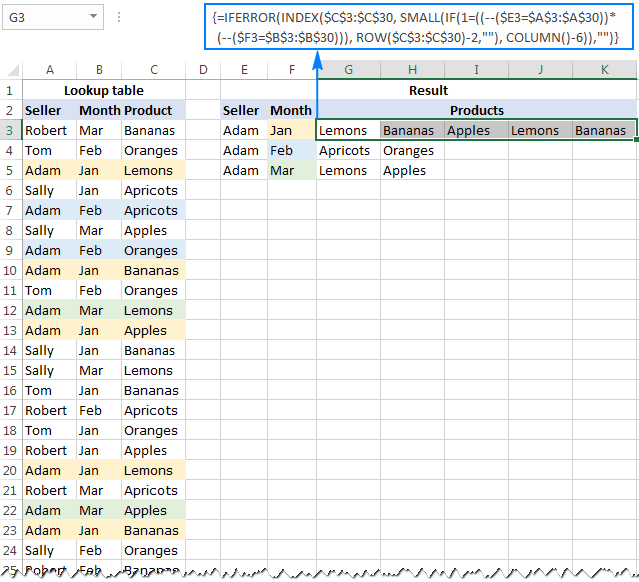In a similar manner, you can do multiple Vlookup with three, four or more conditions.

#### How these formulas work

Basically, the formulas to Vlookup multiple values with multiple conditions work with the already familiar logic, explained in the very first example. The only difference is that the IF function now tests multiple conditions:

1=((--(lookup_value1=lookup_range1))*(--(lookup_value2=lookup_range2))*…)

The result of each lookup_value=lookup_range comparison is an array of logical values TRUE (condition is met) and FALSE (condition is not met). The double unary operator (--) coerces the logical values into 1's and 0's. And because multiplying by zero always gives zero, in the resulting array, you have 1 only for those elements that meet all of the specified conditions. Now, you simply compare the final array with number 1 so that the ROW function returns the numbers of rows that meet all the conditions, an empty string otherwise.

A word of caution. All of the multiple Vlookup formulas discussed in this tutorial are array formulas. As such, each formula iterates through all elements of the arrays every time the source data is changed or the worksheet is recalculated. On large worksheets containing hundreds or thousands of rows, this may significantly slow down your Excel.

If you need to get matches from several sheets, use this guide: How to VLOOKUP across multiple sheets.

## How to Vlookup to return multiple values in one cell

I will be upfront - I don't know an easy way to lookup and return multiple matches in a single sell with formulas. However, I do know a formula-free (read "stress-free" :) way to do this by using two add-ins included with our Ultimate Suite for Excel. The detailed steps follow below.

#### Source data and expected result

As shown in the screenshot, we continue working with the dataset we've used in the previous example. But this time we want to achieve something different - instead of extracting multiple matches in separate cells, we want them to appear in a single sell, separated with a comma, space, or some other delimiter of your choosing.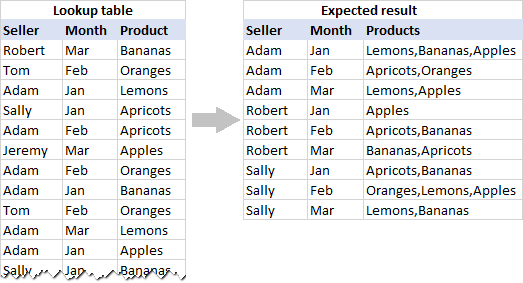#### Pull rows with multiple matches to the main table

In your main table, enter a list of unique names in the first column, months in the second column, and arrange them like shown in the screenshot below. After that, carry out the following steps:

1. Select your main table or click any cell within it, and then click the Merge Two Tables button on the ribbon: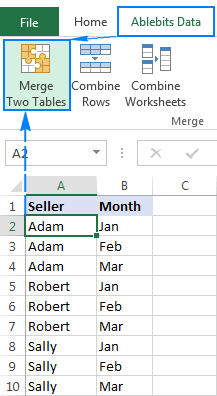2. The add-in is smart enough to identify and pick the entire table, so you just click Next: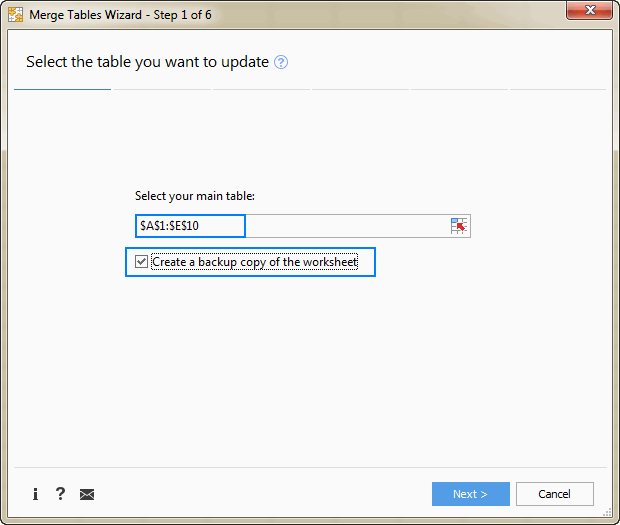Tip. When using the tool for the first time, it stands to reason to select the Create a backup copy of the worksheet box in case something goes wrong.

3. Select the lookup table, and click Next.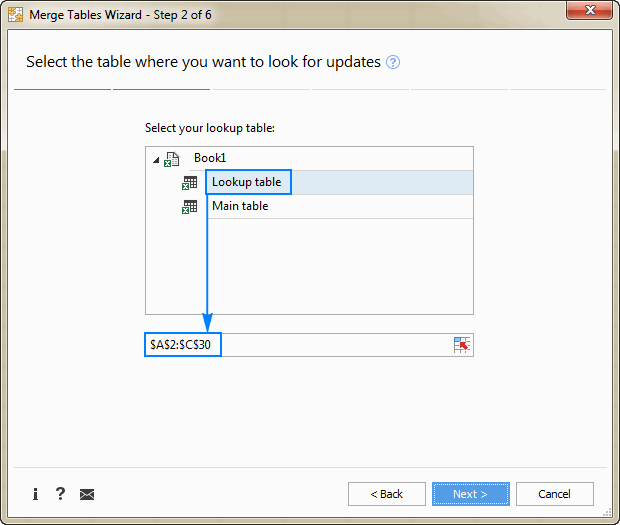4. Choose one or more matching pairs of columns that should be compared in the main table and lookup table (in this example, it's the Seller and Month columns), and then click Next.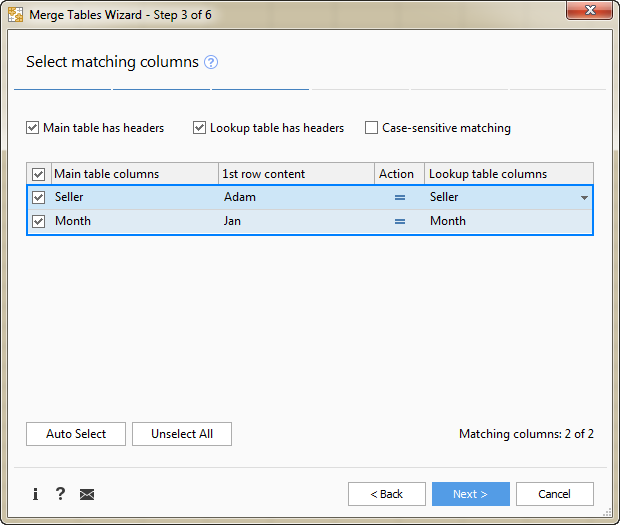5. Select the column(s) from which you want to pull matching values (Product in this example), and click Next.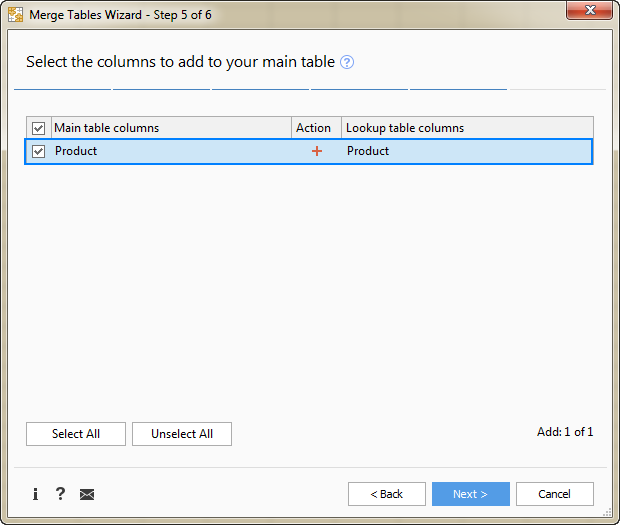6. Tell the add-in how exactly you want multiple matches to be arranged in the main table. For this example, we need the following option: Insert rows with duplicate matching values after the row with the same value. Make sure that no other option is selected and click Finish.At this point, you will have the following result - all matching rows are pulled to the main table and grouped by the values in the lookup columns - first by Seller, and then by Month: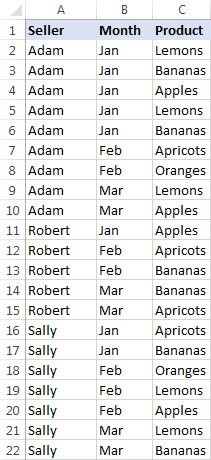The resulting table already looks nice, but it's not exactly what we wanted, right? As you remember, we are looking to Vlookup multiple matches and have them returned in a single sell, comma or otherwise separated.

#### Combine duplicates rows into one row

To merge "duplicate rows" in a single row, we are going to use another tool - Combine Rows Wizard.

1. Select the table produced by the Merge Tables tool (please see the screenshot above) or any cell within the table, and click the Combine Rows button on the ribbon: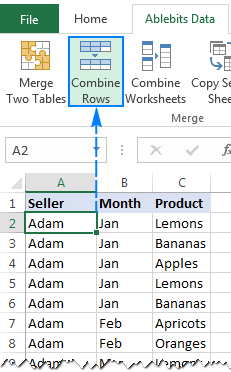2. Check if the add-in's got the table right, and click Next: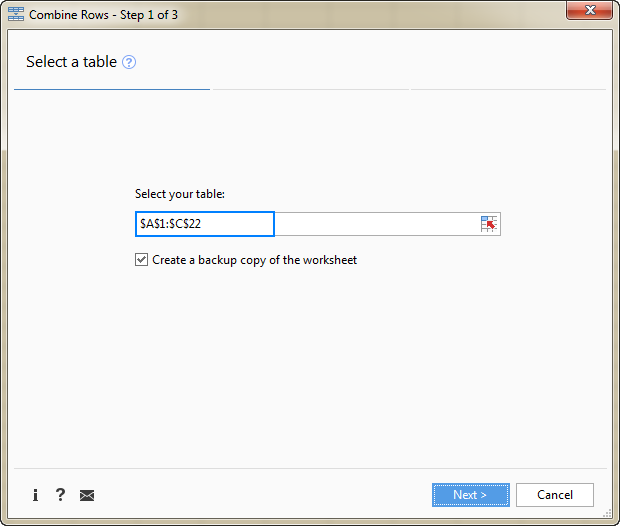3. Select the key column or columns (Seller and Month in this example), and click Next: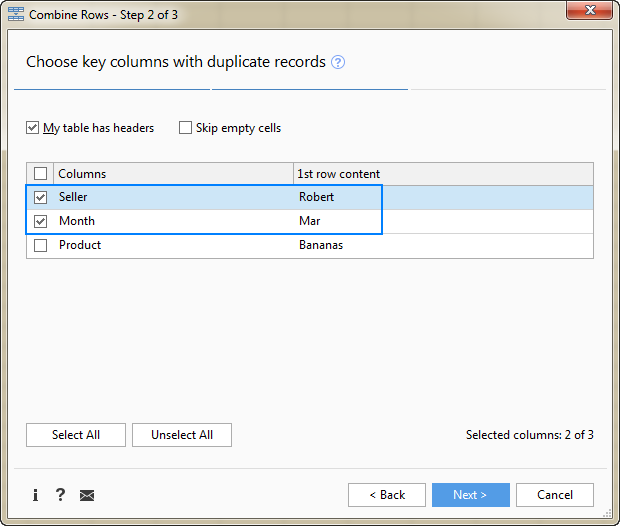4. Select the column(s) that contains multiple matches (Product in this example), choose the desired delimiter (semicolon, comma, space or line break), and click Finish.

Optionally, you can enable one of the additional features, or both:

• Delete duplicate values - if the column to be merged contains a few identical values, the first occurrence will be kept, duplicate matches will be deleted.

In this example, we do not check this option, and the add-in returns all found matches. For example, in cell C2, we have this string: Lemons, Bananas, Apples, Lemons, Bananas (please see the result on step 5 below). If you choose to delete duplicates, the result would be: Lemons, Bananas, Apples.

• Skip empty cells - self-explanatory :)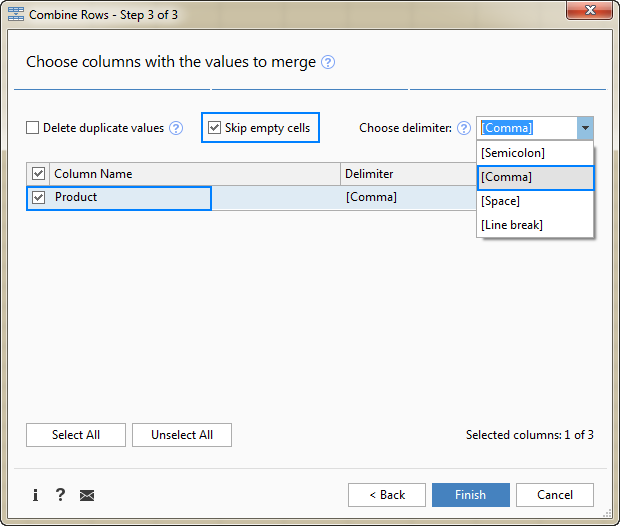5. Allow the add-in a few seconds for processing, and you are all set!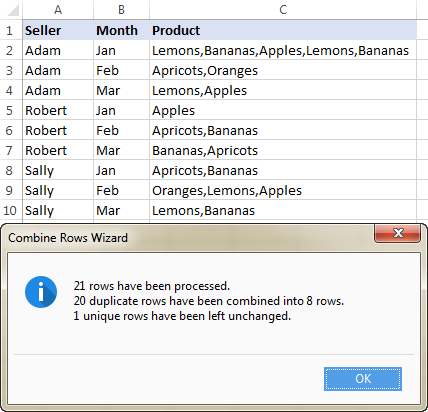This is how you can look up and return multiple values in Excel using our tools. If you are curious to give them a try, a fully-functional evaluation version of the Ultimate Suite is available for download below.

Vlookup Multiple Values - examples (.xlsx file)
Ultimate Suite - trial version (.exe file)

1. I'm Trying To Create a List of all the Sides of a Sheet that get Edging applied to them
So in the A Column I have the Number of Pieces. the B and C Columns Have the Width and Length of the Piece.
The D-G Columns Have the Style of Edging that needs applied to each side. Columns H-K Have the Net Length in Feet Per SIde.
How Do i Create a formula to Pull That Info into a Concise Summary of the total length of each edging required?

• Hi! To understand what you want to do, give an example of the source data and the expected result.

2. Dear Ablebits team,

Thank you for sharing the knowledge.

I use Formula 3. Vlookup multiple matches based on multiple conditions > Return multiple results in a row

I tried to used three conditions, so i add criteria in column D and L, then i use formula :

=IFERROR(INDEX(\$D\$3:\$D\$16,SMALL(IF(1=((--(\$J3=\$A\$3:\$A\$16))*(--(\$K3=\$B\$3:\$B\$16))*(--(\$L3=\$C\$3:\$C\$16))),ROW(\$D\$3:\$D\$16)-2,""),COLUMN()-10)),"")

Unfortunately it's not working, can you help me figure it out?

Thank you

3. Hello,

I am creating a quote reading from a datasheet. one quote number can have different products. What is the formula to show all the products in separate rows. ??

4. Hello,

I have a data set that has over 6000 inputs. I need to pull the data from a cell in a row if it two other cells from the row contain one variable and one nonvariable number. How can i do this?

I am currently using the formula below:

=INDEX('IMDS DATA'!58:9282,MATCH(C263,'IMDS DATA'!E:E,0),22)

however, this list data from the row that "matches" first. I need it to match the row that has both C263 on the E column and "5000" in the V column. How do i do that?

5. How we can get the unique data with this formula, as this one showing duplicate value as well.

6. Hello,

Thanks for the article. I found it very helpful.

Question: Is there a way to Return multiple results in a row without duplication?

7. Hi
How what formula can I use using VLOOKUP for error cell or to blank cell

8. I found this of great help!

I have a question, if the data is in different sheets, will these equations still work? Specifically equation 3.
I managed to make the equation work when the data is in the same sheet, but did not manage when the data was placed in different sheets.

Thanks

PS eq:
worked - data in same sheet
=IFERROR(INDEX(\$F\$2:\$F\$3500, SMALL(IF((--(\$K2=\$B\$2:\$B\$3500)) * (--(\$N2>=\$G\$2:\$G\$3500)) * (--(\$N2=Sheet1!\$G\$2:\$G\$3500)) * (--(\$D2<=Sheet1!\$H\$2:\$H\$3500)), ROW(Sheet1!\$F\$2:\$F\$3500)-1,""), COLUMN()-5)),"")

• /Unfortunately first comment was not published entirely.
Here is the unpublished part/

not worked - return_range in another sheet
Column value subtraction changed to reflect the change in column position.
=IFERROR(INDEX(Sheet1!\$F\$2:\$F\$3500, SMALL(IF((--(\$B2=Sheet1!\$B\$2:\$B\$3500)) * (--(\$D2>=Sheet1!\$G\$2:\$G\$3500)) * (--(\$D2<=Sheet1!\$H\$2:\$H\$3500)), ROW(Sheet1!\$F\$2:\$F\$3500)-1,""), COLUMN()-5)),"")

• Hi!
I can't check the formula because I don't have your data. Try in the COLUMN() function to specify the cell in which the formula is written. For example, COLUMN(F2)